Looking for VBA Tutorials?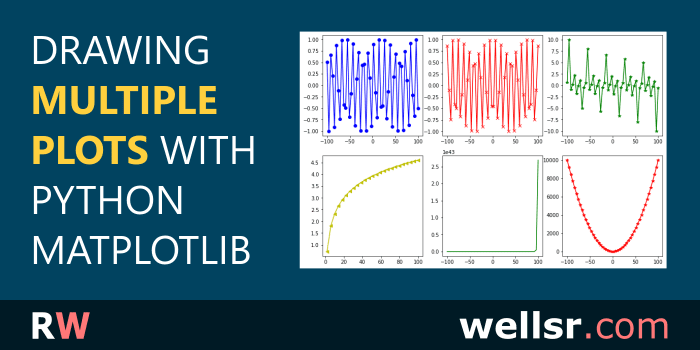#### Nov 19, 2021

In this tutorial, we'll show you exactly how to draw multiple plots at once using the Python Matplotlib library. We're going to study both the subplot() and subplots() functions.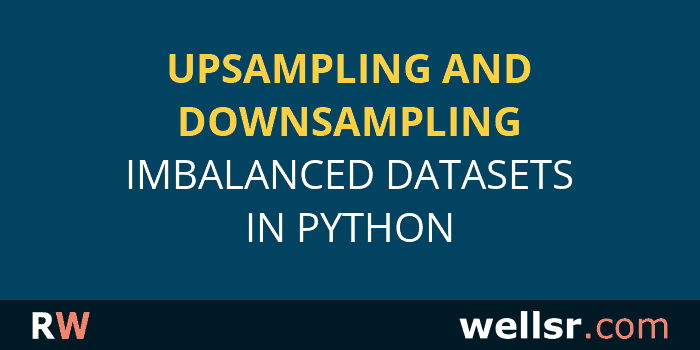#### Nov 12, 2021

Handling imbalanced data in Python is essential. In this tutorial, we'll show you how to balance datasets using two upsampling and one downsampling technique.#### \$120.00 \$57.00

Want to learn more programming languages? We've combined each of our comprehensive VBA reference guides into a single bundle with over 200 tips and macros covering the 125 most important topics in VBA.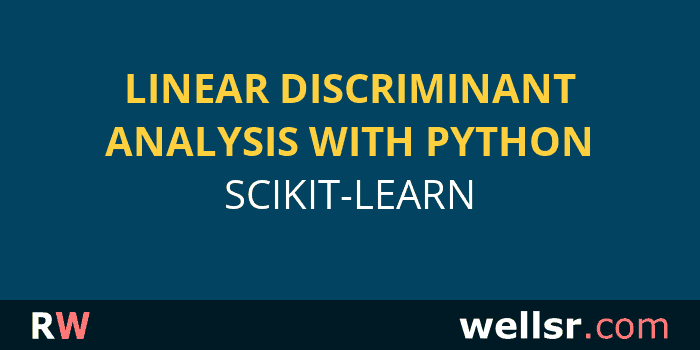#### Oct 29, 2021

Here's how to do linear discriminant analysis (LDA) for dimensionality reduction in Python using sklearn. We'll compare our LDA results to PCA, too.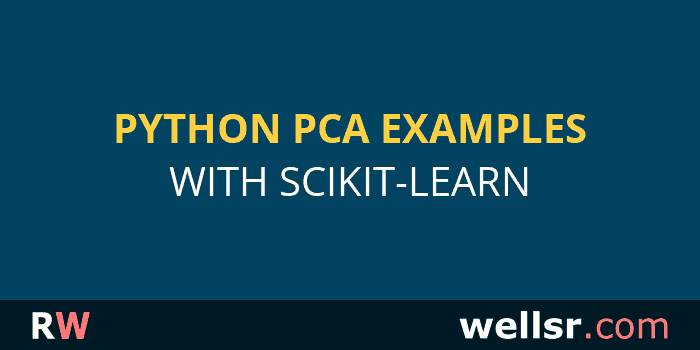#### Oct 22, 2021

Let's take a look at some examples showing how to use principal component analysis (PCA) for dimensionality reduction with the Python scikit-learn library.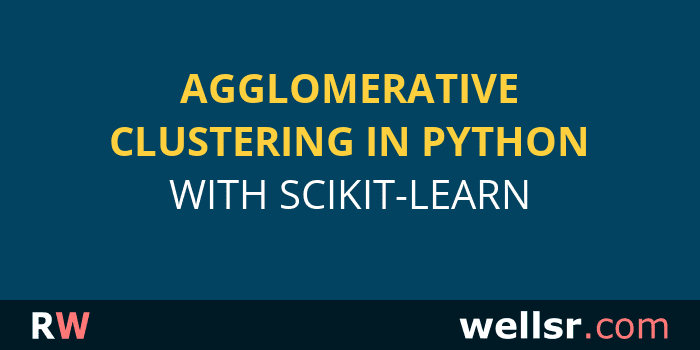#### Oct 15, 2021

We're going to walk through a real-world example of how to perform Python hierarchical clustering in sklearn with the agglomerative clustering algorithm.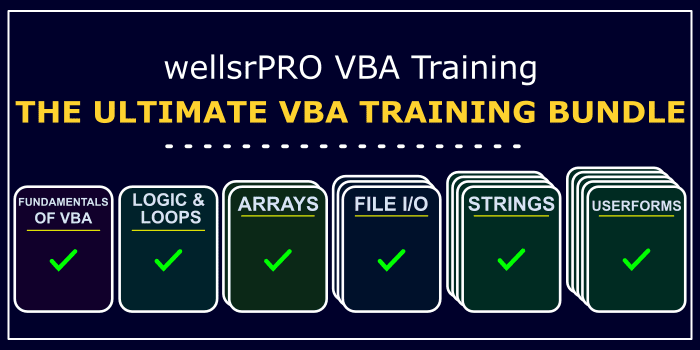#### \$120.00 \$57.00

We created a suite of 6 VBA cheat sheets with over 200 tips showing you everything you need to know to start making power Excel applications. Take a look!#### Oct 8, 2021

Let's talk about how to do asynchronous programming in Python using the asyncio module. Asynchronous functions are functions that execute in parallel.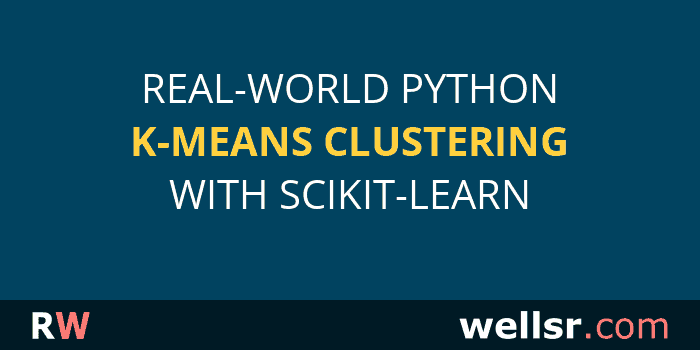#### Sep 24, 2021

Let's walk through a real-world example of how to perform data clustering using the Python scikit-learn k-means clustering algorithm.#### Sep 10, 2021

Today we're going to show you examples of how to calculate the outer product of two vectors or matrices using the NumPy library in Python.#### Aug 13, 2021

Let's learn how to calculate eigenvalues and eigenvectors for a given matrix using the NumPy eigvals and eig methods in Python.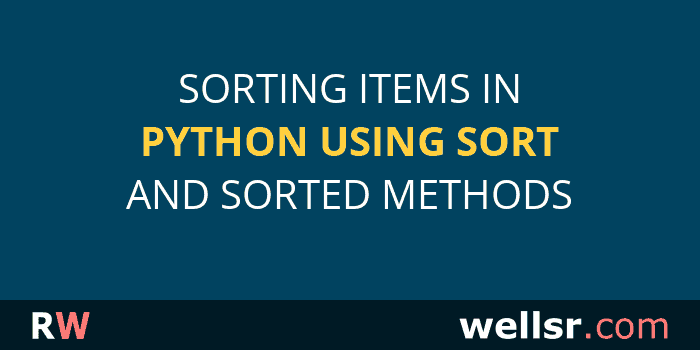#### Jul 31, 2021

This article explains sorting in Python using the Sort and Sorted methods. The former sorts items in-place while the latter create copies of sorted collections, like lists and dictionaries.